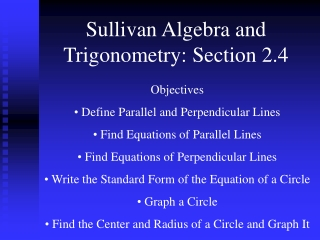DownloadDownload PresentationSullivan Algebra and Trigonometry: Section 2.4

# Sullivan Algebra and Trigonometry: Section 2.4

Télécharger la présentation## Sullivan Algebra and Trigonometry: Section 2.4

- - - - - - - - - - - - - - - - - - - - - - - - - - - E N D - - - - - - - - - - - - - - - - - - - - - - - - - - -
##### Presentation Transcript

1. Sullivan Algebra and Trigonometry: Section 2.4 • Objectives • Define Parallel and Perpendicular Lines • Find Equations of Parallel Lines • Find Equations of Perpendicular Lines • Write the Standard Form of the Equation of a Circle • Graph a Circle • Find the Center and Radius of a Circle and Graph It

2. Definitions: Parallel Lines Two lines are said to be parallel if they do not have any points in common. Two distinct non-vertical lines are parallel if and only if they have the same slope and have different y-intercepts.

3. Find the equation of the line parallel to y = -3x + 5 passing through (1,5). Since parallel lines have the same slope, the slope of the parallel line is m = -3.

4. Definitions: Perpendicular Lines Two lines are said to be perpendicular if they intersect at a right angle. Two non-vertical lines are perpendicular if and only if the product of their slopes is -1.

5. Example: Find the equation of the line perpendicular to y = -3x + 5 passing through (1,5). Slope of perpendicular line:

6. Definition: A circle is a set of points in the xy-plane that are a fixed distance r from a fixed point (h, k). The fixed distance r is called the radius, and the fixed point (h, k) is called the center of the circle. y (x, y) r (h, k) x

7. Definition: The standard form of an equation of a circle with radius r and center (h, k) is

8. 2 2 ( x + 1 ) + ( y - 3 ) = 16 Graph

9. Step 1: Plot the center of the circle. Step 2: Plot points above, below, left, and right of the center by traveling a distance equal to the radius. Step 3: Graph the circle. y (-1, 7) (3,3) (-5, 3) (-1,3) x (-1, -1)

10. The general form of the equation of a circle is: To find the center, radius, and graph of a circle in general form, first rewrite the equation of the circle in standard form using the process of completing the square.